##### Mechanics of Materials For Dummies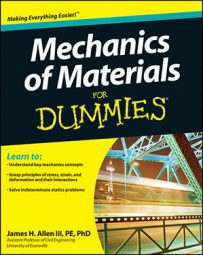Anyone in the mechanical sciences is likely familiar with Mohr's circle — a useful graphical technique for finding principal stresses and strains in materials. Mohr's circle also tells you the principal angles (orientations) of the principal stresses without your having to plug an angle into stress transformation equations.

Starting with a stress or strain element in the XY plane, construct a grid with a normal stress on the horizontal axis and a shear stress on the vertical. (Positive shear stress plots at the bottom.) Then just follow these steps:

1. Plot the vertical face coordinates V(σxx , τxy).

2. Plot the horizontal coordinates H(σyy, –τxy).

You use the opposite sign of the shear stress from Step 1 because the shear stresses on the horizontal faces are creating a couple that balances (or acts in the opposite direction of) the shear stresses on the vertical faces.

3. Draw a diameter line connecting Points V (from Step 1) and H (from Step 2).

4. Sketch the circle around the diameter from Step 3.

The circle should pass through Points V and H as shown here.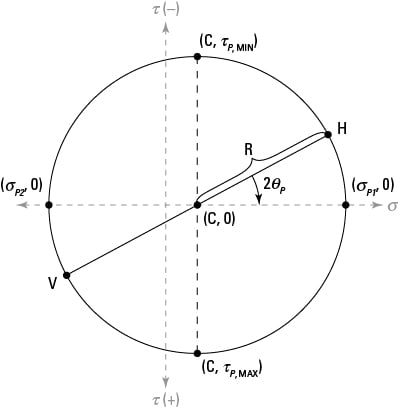5. Compute the normal stress position for the circle's center point (C).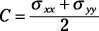6. Calculate the radius (R) for the circle.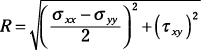7. Determine the principal stresses σP1 and σP2.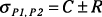8. Compute the principal angles ΘP1 and ΘP2.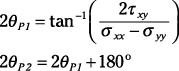You could also use equations directly (instead of Mohr's circle) to determine transformed stresses at any angle: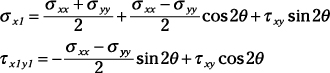To construct a Mohr's circle for strain or to use the transformation equations, substitute εxx for σxx, εyy for σyy, and (0.5)γxy for τxy in the preceding equations.